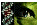Figure 3 of Ryan, Mol Vis 2001; 7:222-227.

Figure 3. Gb 1, 3, and 5 WD repeat profile

The WD repeat profile template was created by Chrysanthe Gaitatzes, Biomolecular Engineering Research Center, Boston University with the series of 7 repeats found in the b1, b3, and b5L sequences of the salamander retina. An x signifies that any amino acid can be found in that position. [0-?] indicates the allowed range of the preceding symbol. Each vertical column represents the set of amino acids that are most frequently found in that position. The over-lines above indicate the position of these strands, with "a" being the strand closest to the central pore and "d" at the external surface of the folded protein. These sheets are arranged around a central pseudosymmetry axis into a beta propeller.

 ```Strand d Strand a Strand b Strand c ___________ ___________ ___________ ___________ x x x x x x x[1-?] G H x x x V x x V x F x x[0-?] P D G[0-3] x L A S G S x D x T I K V W D A I L W S N S I V T A G S V R L F N I L D S P V L S A L I Y C I F I C A V Repeat 1 Beta 1 G H L A K I Y A M H W G T D S R L L V S A S Q D G K L I I W D Beta 3 G H L A K I Y A M H W S N D S R L L V S A S Q D G K L I V W D Beta 5 G H G N K V L C M D W C K D K R R I V S S S Q D G K V I V W D Repeat 2 Beta 1 V H A I P L R S S W V M T C A Y A P S G N Y V A C G G L D N I C S I Y N Beta 3 V H A I P L R S S W V M T C S Y A P S G N F V A C G G L D N M C S I Y N Beta 5 E H A V T M P C T W V M A C A Y A P S G C A I A C G G L D N K C S V Y P Repeat 3 Beta 1 G H T G Y L S C C R F I D D N Q I I T S S G D T T C A L W D Beta 3 A H T G Y L S C C R F L D D N Q I V T S S G D T T C A L W D Beta 5 M H T N Y L S A C S F T N S D M Q I L T A S G D G T C A L W D Repeat 4 Beta 1 G H T G D V M S L S L A P D S R C F V S G A C D A S A K L W D Beta 3 G H T G D C M S L A V S P D F R I F V S G A C D A T A K L W D Beta 5 G H G A D V L C L D L A P S E T G N T F V S G G C D K K A M V W D Repeat 5 Beta 1 G H E S D I N A I C F F P N G N A F A T G S D D A T C R L F D Beta 3 G H E S D I N A I C F F P N G E A V C T G S D D A T C R L F D Beta 5 T H E S D I N S V R Y Y P S G D A F A S G S D D A T C R L F D Repeat 6 Beta 1 S H D N I I C G I T S V A F S K S G R L L L A G Y D D F N C N V W D Beta 3 S H E S I I C G I T S V A F S R S G R L L L A G Y D D F N C N M W D Beta 5 T K E S I I F G A S S V D F S L S G R L L F A G Y N D Y T I N V W D Repeat 7 Beta 1 G H D N R V S C L G V T D D G M A V A T G S W D S F L K I W N Beta 3 G H D N R V S C L G V T T D G M A A A T G S W D S F L K I W N Beta 5 G H E N R V S T L R V S P D G T A F C S G S W D H T L R I W A ```

Ryan, Mol Vis 2001; 7:222-227 <http://www.molvis.org/molvis/v7/a31/>
©2001 Molecular Vision <http://www.molvis.org/molvis/>
ISSN 1090-0535